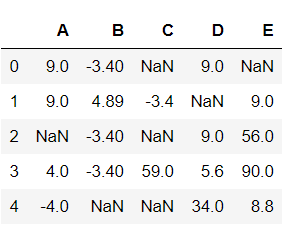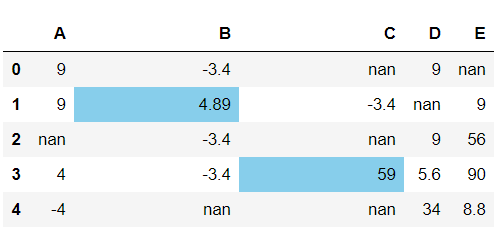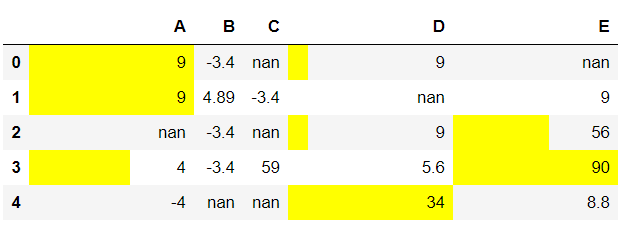Skip to content
Related Articles
How to display bar charts in Pandas dataframe on specified columns?
• Last Updated : 02 Sep, 2020

In this article we will see how to display bar charts in dataframe on specified columns. For doing this task we are using DataFrame.style.bar() method of Pandas Dataframe.

Syntax: pandas.DataFrame.style.bar(columns_list, color)

Return: Dataframe with the given color bar strips on the positive definite values. The None value and the negative values are skipped in the process.

Now, Let’s create a Dataframe:

## Python3

 `# importing required packages``import` `pandas``import` `numpy`` ` `Nan ``=` `numpy.nan`` ` `# creating a dataframe with some nan values``df ``=` `pandas.DataFrame(data ``=` `numpy.array(``                      ``[[``9``, ``-``3.4``, Nan, ``9``, Nan],``                       ``[``9``, ``4.89``, ``-``3.4``, Nan, ``9``],``                       ``[Nan, ``-``3.4``, Nan, ``9``, ``56``],``                       ``[``4``, ``-``3.4``, ``59.0``, ``5.6``, ``90``],``                       ``[``-``4``, Nan, Nan, ``34``, ``8.8``]]),``                       ``columns ``=` `list``(``"ABCDE"``))`` ` `# view dataframe``df`

Output:Example 1:

## Python3

 `# display the bar chart on dataframe``df.style.bar(subset ``=` `[``'B'``, ``'C'``],``                   ``color ``=` `'skyblue'``)`

Output:Example 2:

## Python3

 `# display the bar chart on dataframe``df.style.bar(subset ``=` `[``'A'``, ``'D'``, ``'E'``],``                   ``color ``=` `'yellow'``)`

Output:Attention geek! Strengthen your foundations with the Python Programming Foundation Course and learn the basics.

To begin with, your interview preparations Enhance your Data Structures concepts with the Python DS Course. And to begin with your Machine Learning Journey, join the Machine Learning – Basic Level Course

My Personal Notes arrow_drop_up